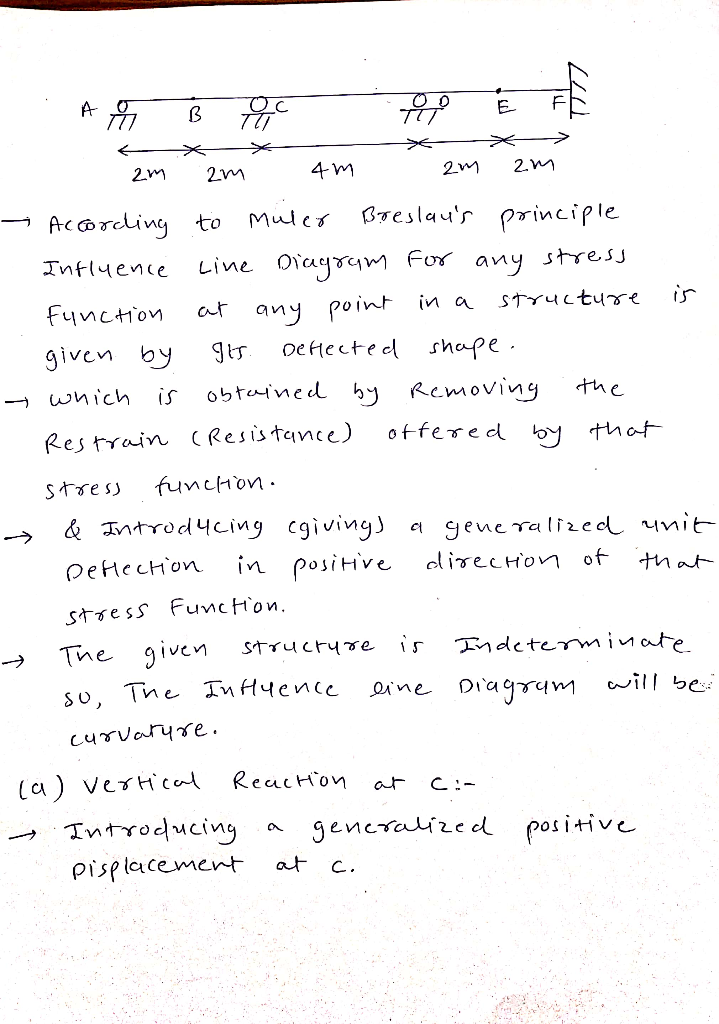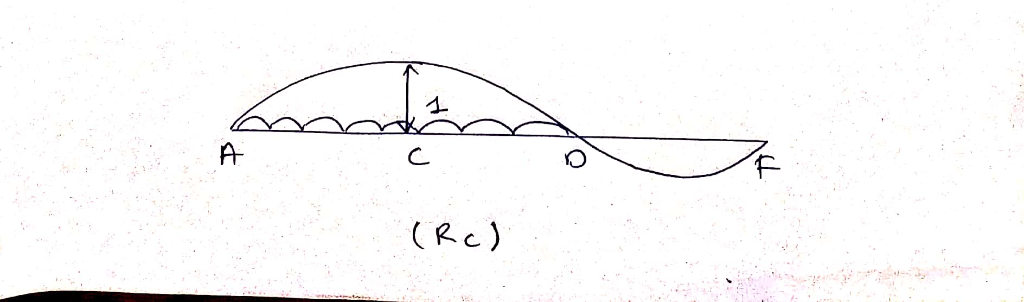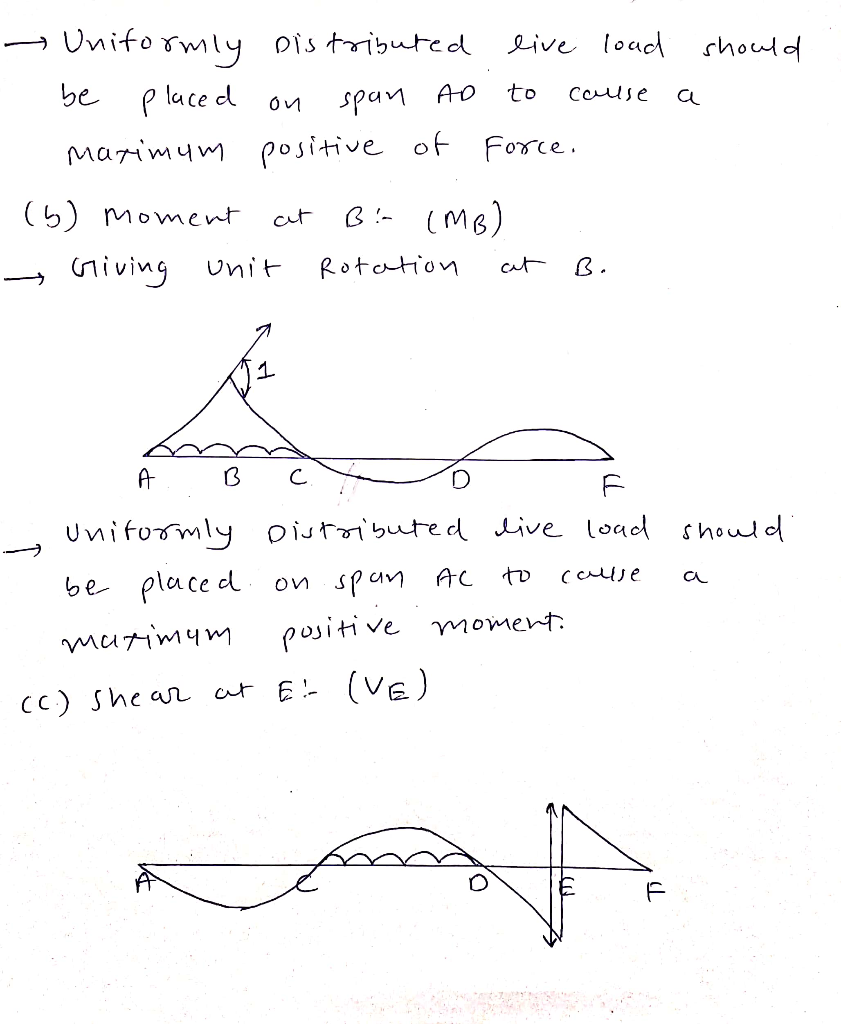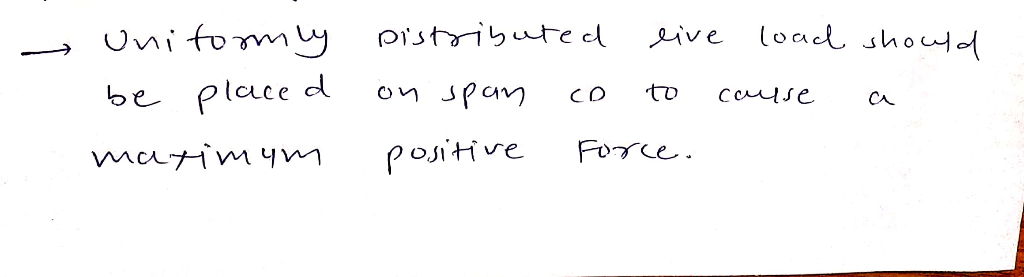Homework Help Question & Answers

Sketch (qualitatively) the influence line for (a) the vertical reaction at C, (b) the moment at B...Sketch (qualitatively) the influence line for (a) the vertical reaction at C, (b) the moment at B, and (c) the shear at E. In each case, indicate on a sketch of the beam where a uniform distributed live load should be placed so as to cause a maximum positive value of the corresponding force/moment investigated. Assume the beam is fixed at F. Supports at A, C, and D are rockers (roller supports) E F 4 m iTmI hope I could help u

Add Answer of: Sketch (qualitatively) the influence line for (a) the vertical reaction at C, (b) the moment at B...
More Homework Help Questions Additional questions in this topic.

• Describe the graph of 3x+y<1 a) a dotted line with shading below the boundary line 3x+y=1 b) a solid line with shading abouve the boundary line 3x+y=1 c) a solild line wtih shading below the boundary line 3x+y=1 d) a dotted line with shading above the

Need Online Homework Help?

Get FREE EXPERT Answers
WITHIN MINUTES
Related Questions